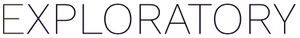# How to automatically number figures in Note

#1

You can number figures automatically in Note using an inline R code. The idea is to create an R variable that holds the current number and increment one after showing each figure. Here is a note example. (somehow just `fignum++` doesn’t work in the inline R code.)

```````r fignum <- 0;`

Picture `r fignum <- fignum+1; fignum;`

Picture `r fignum <- fignum+1; fignum;`

Picture `r fignum <- fignum+1; fignum;`
``````

If you run this note, it will show the following text in the preview.

``````Picture 1

Picture 2

Picture 3
``````

1 Like